• 9863020202
• contact@financeseva.in

# Ratio Analysis Calculator

Financial Statements are prepared by companies to demonstrate its financial activity to stakeholders. These are prepared at regular intervals, and typically contain at least a balance sheet and an income statement. The balance sheet shows the value of a company's accounts at a given point in time. The income statement shows the financial effects of activities over a given period of time.

%
%

%
%
%
%
%
%
%
%
%
%
%
%
%
%

Days
Days

.
.

%
%
%
%

%
%

.
.

%
%

%
%
%

%
%
%

%
%
%
%

%
%
%

%
%
%

%
%

%
%
%
%
%

%
%
%
%
%
%
%

%
%

%
%
%

%

%
%
%
%
%
%

%
%
%

%

%
%
%
%

%

%
%
%
%
%
%
%
%
%
%
%
%
%
%

%
%
%
%

%
%
%
%
%
%
%
%

%
%
%
%
%
%
%
%
%
%
%
%

%
%
%
%
%
%
%
%

%
%
%
%
%
%
%
%
%
%

%
%
%
%
%
%

%
%
%
%
%
%

%
%
%
%
%
%
%
%

%
%
%
%
%
%

### Financial Statement Analysis

#### Liquidity Ratios

##### Current Ratio

The Current Ratio is used to test the company's ability to pay its short term obligations. Below 1 means the company does not have sufficient incoming cash flow to meet its obligations over the coming year.

Current Ratio Formula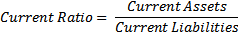Current Ratio Calculator

Use the Current Ratio Calculator above to calculate the current ratio from your financial statements.

##### Quick Ratio

The Quick Ratio is an indicator of a company's short-term liquidity. The quick ratio measures a company's ability to meet its short-term obligations with its most liquid assets. The higher the quick ratio, the better the position of the company.

Quick Ratio Formula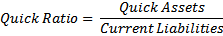Quick Ratio Calculator

Use the Quick Ratio Calculator above to calculate the quick ratio from your financial statements.

#### Profitability Ratios

##### Return on Assets (Profitability Ratio)

Return on Assets is an indicator of how profitable a company is relative to its total assets. ROA gives an idea as to how efficient management is at using its assets to generate earnings.

Return on Assets (Profitability Ratio) Formula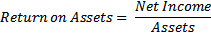Return on Assets (Profitability Ratio) Calculator

Use the Return on Assets (Profitability Ratio) Calculator above to calculate the profitability ratio from your financial statements.

##### Return on Equity

Return on Equity provides the amount of net income returned as a percentage of shareholders equity. Return on equity measures a corporation's profitability by revealing how much profit a company generates with the money shareholders have invested.

Return on Equity FormulaReturn on Equity Calculator

Use the Return on Equity Calculator above to calculate the return on equity from your financial statements.

##### Return on Invested Capital

The Return on Invested Capital measure gives a sense of how well a company is using its money to generate returns. Comparing a company's return on capital (ROIC) with its cost of capital (WACC) reveals whether invested capital was used effectively.

Return on Invested Capital Formula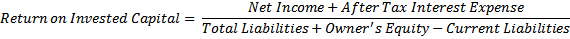Return on Invested Capital Calculator

Use the Return on Invested Capital Calculator above to calculate the return on invested capital from your financial statements

##### Return on Common Equity

Return on Common Equity measures a corporation's profitability by revealing how much profit a company generates with the money shareholders have invested.

Return on Common Equity Formula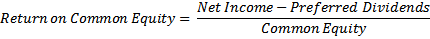Return on Common Equity Calculator

Use the Return on Common Equity Calculator above to calculate the return on common equity from your financial statements.

##### Gross Profit Margin

Gross Profit Margin (Gross Margin) is used to assess a firm's financial health by revealing the proportion of money left over from revenues after accounting for the cost of goods sold.

Gross Profit Margin (Gross Margin) Formula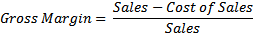Gross Profit Margin (Gross Margin) Calculator

Use the Gross Profit Margin (Gross Margin) Calculator above to calculate the gross profit margin (gross margin) from your financial statements.

##### Profit Margin

Profit Margin is used to determine the profitability of each dollar of sales that company makes.

Profit Margin Formula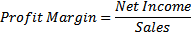Profit Margin Calculator

Use the Profit Margin Calculator above to calculate the profit margin from your financial statements.

##### Operating Margin

Operating Margin shows the profitability of the ongoing operations of the company, before financing expenses and taxes.

Operating Margin Formula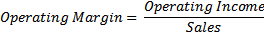Operating Margin Calculator

Use the Operating Margin Calculator to calculate the operating margin from your financial statements.

##### Earnings per Share

Earnings Per Share is the portion of a company's profit allocated to each outstanding share of common stock.

Earnings per Share Formula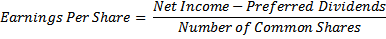Earnings per Share Calculator

Use the Earnings per Share Calculator above to calculate the earnings per share from your financial statements.

#### Activity Analysis

##### Asset Turnover

Asset Turnover measures a firm's efficiency at using its assets to generate sales revenue, the higher the better.

Asset Turnover Formula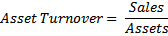Asset Turnover Calculator

Use the Asset turnover calculator above to calculate the asset turnover from your financial statements.

##### Accounts Receivable Turnover

Accounts Receivable Turnover is used to quantify a firm's effectiveness in extending credit as well as collecting debts. The receivables turnover ratio is an activity ratio, measuring how efficiently a firm uses its assets.

Accounts Receivable Turnover Formula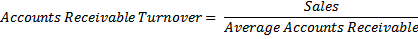Accounts Receivable Turnover Calculator

Use the Accounts Receivable Turnover Calculator to calculate the accounts receivable turnover from your financial statements.

##### Days Receivables

Days Receivables indicates the average number of days that receivables are outstanding. High numbers indicate long collection periods, low numbers indicate efficient collection of receivables.

Days Receivables Formula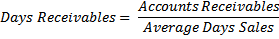Days Receivables Calculator

Use the Days Receivables Calculator to calculate the days receivables from your financial statements.

##### Average Days Sales

Average Days Sales is the average sale per day over the year. This is used for forecasting and to set the expected sales every day over an evenly distributed sales forecast.

Average Days Sales Formula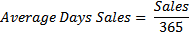Average Days Sales Calculator

Use the Average Days Sales Calculator to calculate the average days sales from your financial statements.

#### Inventory Turnover

Inventory Turnover measures how many times a company's inventory will be sold and replaced in a year.

Inventory Turnover Formula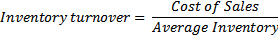Inventory Turnover Calculator

Use the Inventory Turnover Calculator to calculate the inventory turnover from your financial statements.

##### Inventory Turnover Period in Days

Inventory Turnover Period in Days measures how many days it takes for a company to turnover its entire inventory.

Inventory Turnover Period in Days Formula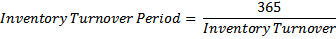Inventory Turnover Period in Days Calculator

Use the Inventory Turnover Period in Days Calculator to calculate the inventory turnover period in days from your financial statements.

##### Fixed Asset Turnover

Fixed Asset Turnover measures the efficiency of fixed assets to generate profit. The higher the number, the more efficient management’s use of fixed assets.

Fixed Asset Turnover Formula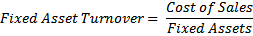Fixed Asset Turnover Calculator

Use the Fixed Asset Turnover Calculator to calculate the fixed asset turnover from your financial statements.

##### Working Capital Turnover

Working Capital Turnover measures the depletion of working capital to the generation of sales over a given period. This provides some useful information as to how effectively a company is using its working capital to generate sales.

Working Capital Turnover Formulas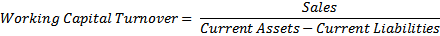Working Capital Turnover Calculator

Use the Working Capital Turnover Calculator above to calculate the working capital turnover from you financial statements.

#### Capital Structure Analysis

##### Debt Ratio

The Debt Ratio indicates what proportion of debt a company has relative to its assets. The measure gives an idea to the leverage of the company along with the potential risks the company faces in terms of its debt-load.

Debt Ratio Formula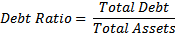Debt Ratio Calculator

Use the Debt Ratio Calculator to calculate the debt ratio from your financial statements.

##### Debt to Equity Ratio

The Debt to Equity Ratio is a measure of a company's financial leverage. It indicates what proportion of equity and debt the company is using to finance its assets.

Debt to Equity Ratio Formula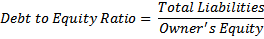Debt to Equity Ratio Calculator

Use the Debt to Equity Ratio Calculator above to calculate the debt to equity ratio form your financial statements

##### Debt to Tangible Net Worth

The Debt to Tangible Net Worth Ratio is a measure of a company's financial leverage to the tangible asset value of owner's equity. It indicates what proportion of equity and debt the company is using to finance its tangible assets.

Debt to Tangible Net Worth Formula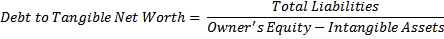Debt to Tangible Net Worth Calculator

Use the Debt to Tangible Net Worth Calculator above to calculate the debt to tangible net worth from your financial statements.

##### Times Interest Earned

Times Interest Earned is used to measure a company's ability to meet its debt obligations. Failing to meet these obligations could force a company into bankruptcy.

Times Interest Earned Formula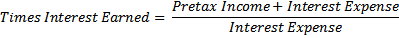Times Interest Earned Calculator

Use the Times Interest Earned Calculator above to calculate the times interest earned from you financial statements.

##### Debt Servicing Ratio

Debt Servicing Ratio is used to assess a company’s ability to meet all of its debt repayment obligations, both interest and principal repayments.

Debt Servicing Ratio Formula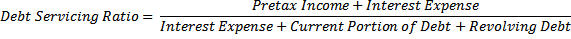Debt Servicing Ratio Calculator

Use the Debt Servicing Ratio Calculator above to calculate the debt servicing ratio from your financial statements.

#### Market Analysis

##### Price to Earnings Ratio

The Price to Earnings Ratio tells us how many years it will take for earnings to repay the current market share price. This is a useful measure to compare companies in the same industry.

Price to Earnings Ratio Formula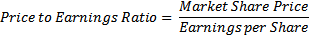Price to Earnings Ratio Calculator

Use the Price to Earnings Ratio Calculator above to calculate the price to earnings ratio from your financial statements.

##### Dividend Yield

The Dividend Yield shows how much a company pays out in dividends each year relative to its share price. In the absence of any capital gains, the dividend yield is the return on investment for a stock.

Dividend Yield Formula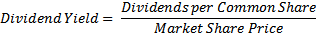Dividend Yield Calculator

Use the Dividend Yield Calculator above to calculate the dividend yield from your financial statements

##### Dividend Payout Ratio

The Dividend Payout Ratio is the percentage of earnings that are paid out to shareholders. Earnings not paid to shareholders are expected to be retained by the company and invested in further operations.

Dividend Payout Ratio Formula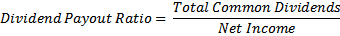Dividend Payout Ratio Calculator

Use the Dividend Payout Ratio Calculator above to calculate the dividend payout ratio from your financial statements.

##### Price to Book Ratio

Price to Book Ratio tells us the relative value the market places on the company to the accounting valuation. This ratio provides a basic understanding of residual value of a company should it go bankrupt.

Price to Book Ratio Formula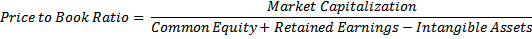Price to Book Ratio Calculator

Use the Price to Book Ratio Calculator to calculate the price to book ratio from your financial statements.

##### Earnings per Share

Earnings Per Share is the portion of a company's profit allocated to each outstanding share of common stock.

Earnings per Share FormulaEarnings per Share Calculator

Use the Earnings per Share Calculator above to calculate the earnings per share from your financial statements.

##### Du Pont Analysis

Du Pont Analysis is used to identify the components of business operations that lead to shareholders return. Total return on equity is the profitability, multiplied by the rate of asset turnover, multiplied by the ratio of assets to equity (leverage). By identifying each component and evaluating, strength and weakness can be evaluated, as well as insight into competitive advantage. Understanding how each element leads to return on equity will help a researcher investigate further into the operations of a company.

Du Pont Analysis Formula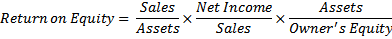Du Pont Analysis Calculator

Use the Du Pont Analysis Calculator above to calculate the Du Pont Ratios from your financial statements.

##### Profit Margin (Du Pont)

Profit Margin (Du Pont) is used to determine the profitability of each dollar of sales that company makes.

Profit Margin Formula (Du Pont)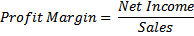Profit Margin (Du Pont) Calculator

Use the Profit Margin (Du Pont) Calculator above to calculate the profit margin and Du Pont ratios from your financial statements.

##### Asset Turnover (Du Pont)

Asset Turnover (Du Pont) measures a firm's efficiency at using its assets to generate sales revenue, the higher the better.

Asset Turnover (Du Pont) FormulaAsset Turnover (Du Pont) Calculator

Use the Asset Turnover (Du Pont) Calculator to calculate the asset turnover and Du Pont ratios from your financial statements.

##### Leverage of Assets

Leverage of Assets measures the ratio between assets and owner's equity of a company. The higher the number, the higher the leverage.

Leverage of Assets Formula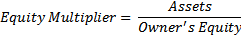Leverage of Assets Calculator

Use the Leverage of Assets Calculator above to calculate the leverage of assets and Du Pont ratios from your financials statements.

##### Analysis of Leverage

Analysis of Leverage is used to evaluate how effectively management is using borrowed funds to make a return for income. Typically, funds are raised by debt in order to enhance the return to shareholders. This is done by financing the company’s assets with debt, which requires a fixed payment of interest. If the assets financed by debt generate pretax net income sufficient to repay this interest, then any additional net income is profit that goes to the shareholders.

A company’s assets can be divided into assets funded by equity, and assets funded by debt. It is possible to analyze the efficiency with which a company’s assets generate pretax income, and allocate this income in proportion to the capital structure. We can then determine the amount that each set of assets contributes to net income. We would expect that management would be able to use assets financed by debt to generate enough net income to pay the borrowing costs, and hopefully produce additional income for the shareholders. If the income generated by the borrowed assets is negative, then it may be advisable for a company to alter its capital structure, or focus on improving the efficiency of its assets in regards to generating net income.

Gross Efficiency of Assets tells us how much income each dollar of assets generates before paying out taxes and interest. This tells us how efficiently management uses its assets.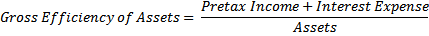Pretax Income is a made up of two sources, income from assets funded by shareholders equity, and assets funded by borrowed debt.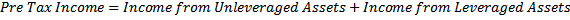Income from Unleveraged Assets is the income generated by the assets funded by shareholders equity and operations.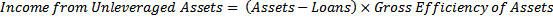Income from Leveraged Assets is the income generated by assets funded by borrowed debt.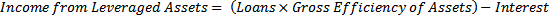Leveraged Assets Contribution to NI is the percentage of the pretax income that is provided by management’s use of debt to fund assets. Higher numbers show good use of debt. Negative number show losses generated by the assets financed by debt.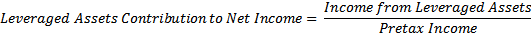##### Sustainable Growth Rate

Sustainable Growth Rate is the maximum growth rate of a company if none of its ratios change and it does not raise new capital through selling shares.

Sustainable Growth Rate Formula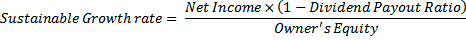Sustainable Growth Rate Calculator

Use the Sustainable Growth Rate Calculator to calculate the sustainable growth rate from your financial statements.CA Vikas Jain - Chief Advisor

Mr. Vikas Jain is a reputed Chartered Accountant possessing in-depth understand...